# How to use the Excel COS function

COS function is a Mathematical function to calculate COS of the angle of the triangle.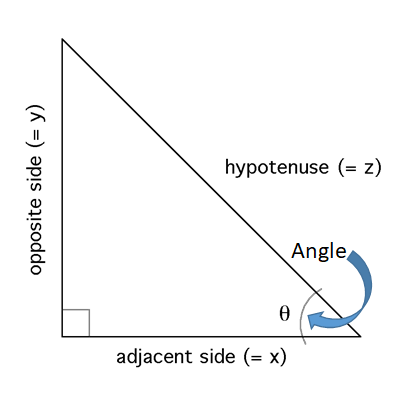COS of an angle is the angle made by the side opposite to the angle and hypotenuse
COS(Angle()) = opposite side(=y)or base / hypotenuse(=z)
Syntax:

=COS (number)

Number in radians or use Radians function to convert degrees to radians OR multiply the angle(in degrees) with PI()/180

Let’s understand this function using it an example.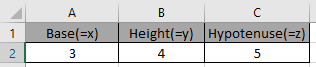These are the lengths of the Triangle having base, height and hypotenuse.

So we need to find the COS of the Angle.
Use the formula:

=COS(B2/C2)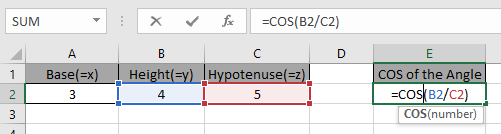Press Enter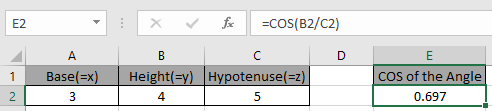As you can see the COS of the angle of the triangle.

The above method was for having sides of the triangle. If you have the angle in degrees and you need to find the COS of that angle.

Use the formula:

It will get you the COS of the 30 degrees angle.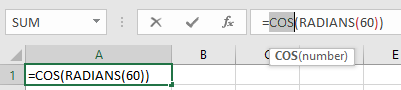Press Enter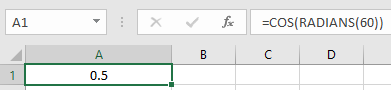Using PI()/180 method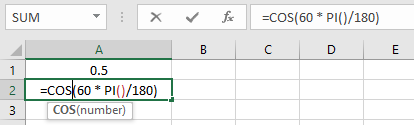Press Enter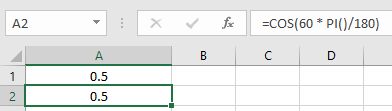As you can see the Cos of the angle using COS function.

Hope you understood How to use COS function in Excel. Explore more articles on Mathematical function like SIN and TAN here. Please state your query in the comment box below. We will assist you.

Related Articles:

How to use the SIN Function in Excel

How to use the SINH Function in Excel

How to use the COSH Function in Excel

How to use the Excel TAN function

How to use the TANH Function in Excel

Popular Articles:

50 Excel Shortcuts to Increase Your Productivity

How to use the VLOOKUP Function in Excel

How to use the COUNTIF function in Excel 2016

How to Use SUMIF Function in Excel

Terms and Conditions of use

The applications/code on this site are distributed as is and without warranties or liability. In no event shall the owner of the copyrights, or the authors of the applications/code be liable for any loss of profit, any problems or any damage resulting from the use or evaluation of the applications/code.